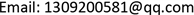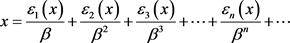﻿ β-连分数的渐近分数 The Convergent in Continued β-Fraction

Vol. 07  No. 12 ( 2018 ), Article ID: 28261 , 5 pages
10.12677/AAM.2018.712192

The Convergent in Continued β-Fraction

Qian Xiao

South China University of Technology, Guangzhou GuangdongReceived: Dec. 2nd, 2018; accepted: Dec. 21st, 2018; published: Dec. 28th, 2018ABSTRACT

Let $\beta =\frac{\sqrt{5}+1}{2}$ , T is the continued β-fraction transformation on [0,1), and $\frac{{p}_{n}\left(x\right)}{{q}_{n}\left(x\right)}$ is the nth-order β-fraction-convergent of x. In this paper, we show many properties of the sequence ${\left(\frac{{p}_{n}\left(x\right)}{{q}_{n}\left(x\right)}\right)}_{n\ge 1}$ . Moreover, we prove that ${\left(\frac{{p}_{n}\left(x\right)}{{q}_{n}\left(x\right)}\right)}_{n\ge 1}$ converges to x.

Keywords:Continued β-Fraction, Convergent, Convergesβ-连分数的渐近分数$\beta =\frac{\sqrt{5}+1}{2}$ ，T为[0,1)上的β-连分数变换， $\frac{{p}_{n}\left(x\right)}{{q}_{n}\left(x\right)}$ 是x的n阶β-渐近分数。本文证明了序列 ${\left(\frac{{p}_{n}\left(x\right)}{{q}_{n}\left(x\right)}\right)}_{n\ge 1}$ 的一些性质，并且证明了 ${\left(\frac{{p}_{n}\left(x\right)}{{q}_{n}\left(x\right)}\right)}_{n\ge 1}$ 收敛且收敛到x。1. 引言

${T}_{\beta }\left(x\right)=\beta x-⌊\beta x⌋=\left\{\beta x\right\}$ , $x\in \left[0,1\right)$ ,${ℤ}_{\beta }^{+}=\left\{所有的非负\beta -整数\right\}=\left\{\xi :\exists x\ge 0,\text{\hspace{0.17em}}s.t.\text{\hspace{0.17em}}\xi ={⌊x⌋}_{\beta }\right\}$

$\beta =\frac{\sqrt{5}+1}{2}$ 时，我们知道

${ℤ}_{\beta }^{+}=\left\{m+n\beta |m,n\in ℤ,m,n\ge 0,-1  。

$T\left(0\right):=0$ , $T\left(x\right)=\frac{1}{x}-{⌊\frac{1}{x}⌋}_{\beta }$ , $x\in \left(0,1\right)$ ,

$x={⌊x⌋}_{\beta }+\frac{1}{{⌊\frac{1}{x}⌋}_{\beta }+\frac{1}{{⌊\frac{1}{T\left(x\right)}⌋}_{\beta }+\cdots +\frac{1}{{⌊\frac{1}{{T}^{K-1}\left(x\right)}⌋}_{\beta }+{T}^{k}\left(x\right)}}}:=\left[{a}_{0}\left(x\right);{a}_{1}\left(x\right),{a}_{2}\left(x\right),\cdots ,{a}_{k}\left(x\right)+{T}^{k}\left(x\right)\right]$ ,

$={⌊x⌋}_{\beta }+\frac{1}{{⌊\frac{1}{x}⌋}_{\beta }+\frac{1}{{⌊\frac{1}{T\left(x\right)}⌋}_{\beta }+\cdots }}:=\left[{a}_{0}\left(x\right);{a}_{1}\left(x\right),{a}_{2}\left(x\right),\cdots \right]$ ,

2. 定理及证明

${p}_{n}={a}_{n}{p}_{n-1}+{p}_{n-2}$

${q}_{n}={a}_{n}{q}_{n-1}+{q}_{n-2}\ge 2{q}_{n-2}\ge \cdots \ge {2}^{\frac{n-2}{2}}$

${p}_{n}{q}_{n-1}-{q}_{n}{p}_{n-1}={\left(-1\right)}^{n-1}$

${p}_{n}{q}_{n-1}={a}_{n}{p}_{n-1}{q}_{n-1}+{p}_{n-2}{q}_{n-1}$

${q}_{n}{p}_{n-1}={a}_{n}{q}_{n-1}{p}_{n-1}+{q}_{n-2}{p}_{n-1}$

$\frac{{p}_{n}}{{q}_{n}}-\frac{{p}_{n-1}}{{q}_{n-1}}=\frac{{\left(-1\right)}^{n-1}}{{q}_{n}{q}_{n-1}}$

$\frac{{p}_{n}}{{q}_{n}}-\frac{{p}_{n-1}}{{q}_{n-1}}=\frac{{\left(-1\right)}^{n-1}}{{q}_{n}{q}_{n-1}}$

${q}_{n}{p}_{n-2}-{p}_{n}{q}_{n-2}={\left(-1\right)}^{n-1}{a}_{n}$

${p}_{n}{q}_{n-2}={a}_{n}{p}_{n-1}{q}_{n-2}+{p}_{n-2}{q}_{n-2}$

${q}_{n}{p}_{n-2}={a}_{n}{q}_{n-1}{p}_{n-2}+{q}_{n-2}{p}_{n-2}$

$\frac{{p}_{n-2}}{{q}_{n-2}}-\frac{{p}_{n}}{{q}_{n}}=\frac{{\left(-1\right)}^{n-1}{a}_{n}}{{q}_{n}{q}_{n-2}}$

$\frac{{p}_{n-2}}{{q}_{n-2}}-\frac{{p}_{n}}{{q}_{n}}=\frac{{\left(-1\right)}^{n-1}{a}_{n}}{{q}_{n}{q}_{n-2}}$

$\begin{array}{c}\frac{{p}_{n}}{{q}_{n}}=\frac{{p}_{n-1}}{{q}_{n-1}}+\frac{{\left(-1\right)}^{n-1}}{{q}_{n}{q}_{n-1}}=\frac{{p}_{n-2}}{{q}_{n-2}}+\frac{{\left(-1\right)}^{n-2}}{{q}_{n-1}{q}_{n-2}}+\frac{{\left(-1\right)}^{n-1}}{{q}_{n}{q}_{n-1}}=\cdots \\ =\frac{{p}_{0}}{{q}_{0}}+\frac{1}{{q}_{0}{q}_{1}}+\frac{-1}{{q}_{1}{q}_{2}}+\cdots +\frac{{\left(-1\right)}^{n-1}}{{q}_{n}{q}_{n-1}}={a}_{0}+\frac{1}{{q}_{0}{q}_{1}}+\frac{-1}{{q}_{1}{q}_{2}}+\cdots +\frac{{\left(-1\right)}^{n-1}}{{q}_{n}{q}_{n-1}}\end{array}$

$x=\frac{{p}_{n}\left(x\right)+{p}_{n-1}\left(x\right){T}^{n}\left(x\right)}{{q}_{n}\left(x\right)+{q}_{n-1}\left(x\right){T}^{n}\left(x\right)}$The Convergent in Continued β-Fraction[J]. 应用数学进展, 2018, 07(12): 1645-1649. https://doi.org/10.12677/AAM.2018.712192

1. 1. Rényi, A. (1957) Representations for Real Numbers and Their Ergodic Properties. Acta Mathematica Academiae Sci-entiarum Hungaricae, 8, 477-493. https://doi.org/10.1007/BF02020331

2. 2. Li, B. and Wu, J. (2008) Be-ta-Expansion and Continued Fraction Expansion. Journal of Mathematical Analysis and Applications, 339, 1322-1331.

3. 3. Parry, W. (1960) On the β-Expansions of Real Numbers. Acta Mathematica Academiae Scientiarum Hungaricae, 11, 401-416. https://doi.org/10.1007/BF02020954

4. 4. Bertrand, A. (1977) Développements en base de Pisot et répartition modulo 1, C. R. Acad. Sci. Paris Sér. A-B 285. No. 6, A419-A421.

5. 5. Schmidit, K. (1980) On Periodic Expansions of Pisot Numbers and Salem Numbers. Bulletin of the London Mathematical Society, 12, 269-278.

6. 6. Gazeau, J.P. and Krejcar, R. (1998) Beta-Integer as Natural Counting Systems for Quasicrystals. Journal of Physics A, 31, 6449-6472.

7. 7. Xiao, Q., Ma, C. and Wang, S.L. On the Eventually Periodic Continued β-Fractions and Their Lévy Constants.